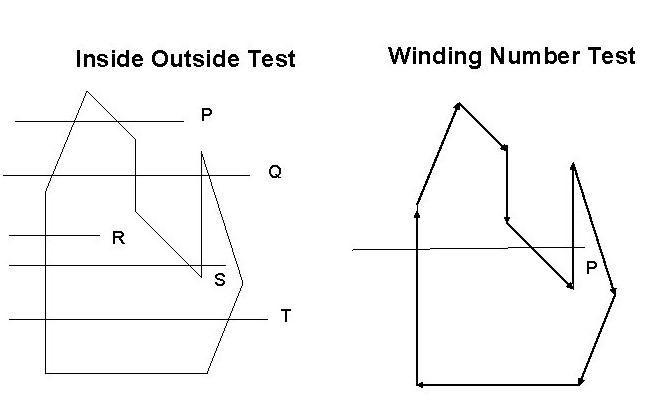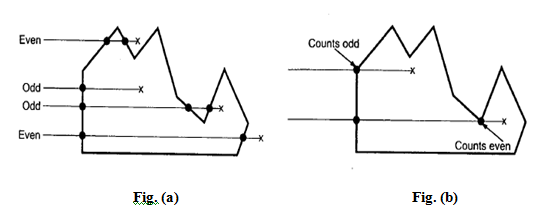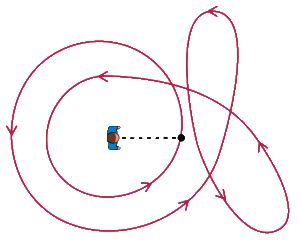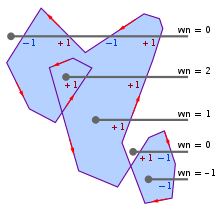×

Search anything:

# Inside Outside Test [2 algorithms: Even-Odd and Winding Number]

#### Algorithms computational geometry computer graphicsIn Computer Graphics, Inside Outside is performed to test whether a given point lies inside of a closed polygon or not. Mainly, there are two methods to determine a point is interior/exterior to polygon:

1. Even-Odd / Odd-Even Rule or Odd Parity Rule
2. Winding Number Method## Even-Odd Rule / Odd Parity Rule

It is also known as crossing number and ray casting algorithm. The algorithm follows a basic observation that if a ray coming from infinity crosses through border of polygon, then it goes from outside to inside and outside to inside alternately. For every two crossings, point lies outside of polygon.

Algorithm:

1. Construct a line segment from point to be examined to point outside of a polygon.
2. Count the number of intersections of line segment with polygon boundaries.
3. If Odd number of intersection, then Point lies inside of Polygon.
4. Else, Point lies outside of polygon.The pseudocode is as follows:

``````count = 0
foreach side in polygon:
if line_intersect_side(P,side):
count = count + 1
if count % 2 == 1:
return inside
else
return outside
``````

This test fails in case line segment intersects at vertex point. To handle it, few modifications are made. Look at other end points of two line segments of polygon.

• If end points lie is at same side of constructed line segment, then even number of intersection is considered for that intersection point.
• If end points lie at opposite side of it, then odd number of intersection is considered.

Example
In Fig.(a) , it can be seen that points having total odd number of intersections lies inside the polygon and vice-versa. In Fig.(b) representing special case, odd/even count is taken according to side orientation.

Sample Implementation of Even-Odd Rule / Odd Parity Rule:

``````// crossing number and ray casting algorithm
// iq.opengenus.org
import static java.lang.Math.*;

public class RayCasting {

static boolean intersects(int[] A, int[] B, double[] P) {
if (A > B)
return intersects(B, A, P);

if (P == A || P == B)
P += 0.0001;

if (P > B || P < A || P >= max(A, B))
return false;

if (P < min(A, B))
return true;

double red = (P - A) / (double) (P - A);
double blue = (B - A) / (double) (B - A);
return red >= blue;
}

static boolean inside_out(int[][] shape, double[] pnt) {
boolean inside = false;
int len = shape.length;
for (int i = 0; i < len; i++) {
if (intersects(shape[i], shape[(i + 1) % len], pnt))
inside = !inside;
}
return inside;
}

public static void main(String[] a) {
double[][] testPoints = {{10, 10}, {10, 16}, {-20, 10}, {0, 10},
{20, 10}, {16, 10}, {20, 20}};

for (int[][] shape : shapes) {
for (double[] pnt : testPoints)
System.out.printf("%7s ", inside_out(shape, pnt));
System.out.println();
}
}

final static int[][] square = {{0, 0}, {20, 0}, {20, 20}, {0, 20}};

final static int[][] hexagon = {{6, 0}, {14, 0}, {20, 10}, {14, 20},
{6, 20}, {0, 10}};

final static int[][][] shapes = {square, hexagon};
}
``````

Time Complexity:

• O(S) where S is the number of sides in the polygon.

# Winding Number / Non-Zero Algorithm

Alternative algorithm to perform test is Winding Number algorithm. A winding Number is calculated for given point with respect to polygon. If winding number is non-zero, then point lies inside the polygon. Else, it lies outside of polygon.

Calculation of Winding Number

Conceptually,to check a point P, construct a line segment starting from P to point on boundary.Treat line segment to be elastic pinned at P. Stretch other end of elastic around the polygon for one complete cycle. Check how many times elastic has been wounded around point P. If count is non-zero, then point lies inside of polygon. Else, outside of polygon.Another way to score up winding number is to assign a score for each intersection with boundary of polygon and sum these numbers. The score is given by considering direction of edge of polygon with respect to line segment constructed. Hence, directions are assigned to each edge of polygon in counter-clock manner. If side of edge starts from below of constructed line, then score -1 is given. If edge starts from above of constructed line then score 1 is given. Else, score 0 is given.

Sample implementation:

``````# Winding Number / Non-Zero Algorithm
# iq.opengenus.org
def winding_number_util(P, V):
winding_number = 0   # the winding number counter

# repeat the first vertex at end
V = tuple(V[:]) + (V,)

# loop through all edges of the polygon
# edge from V[i] to V[i+1]
for i in range(len(V)-1):
# start y <= P
if V[i] <= P:
# an upward crossing
if V[i+1] > P:
# P left of edge
if is_left(V[i], V[i+1], P) > 0:
# have a valid up intersect
winding_number += 1
# start y > P (no test needed)
else:
# a downward crossing
if V[i+1] <= P:
# P right of edge
if is_left(V[i], V[i+1], P) < 0:
# have a valid down intersect
winding_number -= 1
return wn
``````

Time Complexity:

• O(S) where S is the number of sides in the polygon.Example
For a given figure,
1. For a top-most point, Winding number = (-1) + (1) + (-1) + (1) = 0 , lies outside.
2. For bottom-most point, Winding number = -1 , lies inside.

With this article at OpenGenus, you must have a good idea of Inside Outside Tests. Enjoy.